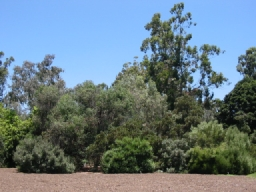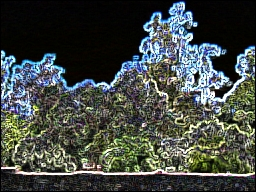# Prewitt Edge

The Prewitt Edge filter is use to detect edges based applying a horizontal and verticle filter in sequence. Both filters are applied to the image and summed to form the final result. The two filters are basic convolution filters of the form:

Horizontal FilterVerticle Filter
 1 1 1 0 0 0 -1 -1 -1
 -1 0 1 -1 0 1 -1 0 1

For example, if a 3x3 window is used as such

 p1 p2 p3 p4 p5 p6 p7 p8 p9
where the filter is centered on p5 with p4 being pixel[x-1][y] and p6 being pixel[x+1][y], etc. then the forumla to calculate the resulting new p5 pixel is

pixel = (p1+p2+p3-p7-p8-p9)+(p3+p6+p9-p1-p4-p7)

which is them clamped to the 0-255 range.

Note that the actual forumla uses the horizontal and verticle components into the final form

pixel = SQRT((X*X)+(Y*Y))

where X = (p1+p2+p3-p7-p8-p9) and Y = (p3+p6+p9-p1-p4-p7)

but for performance reasons we approximate the result and leave the final formula out.

## Example

 Source Prewitt